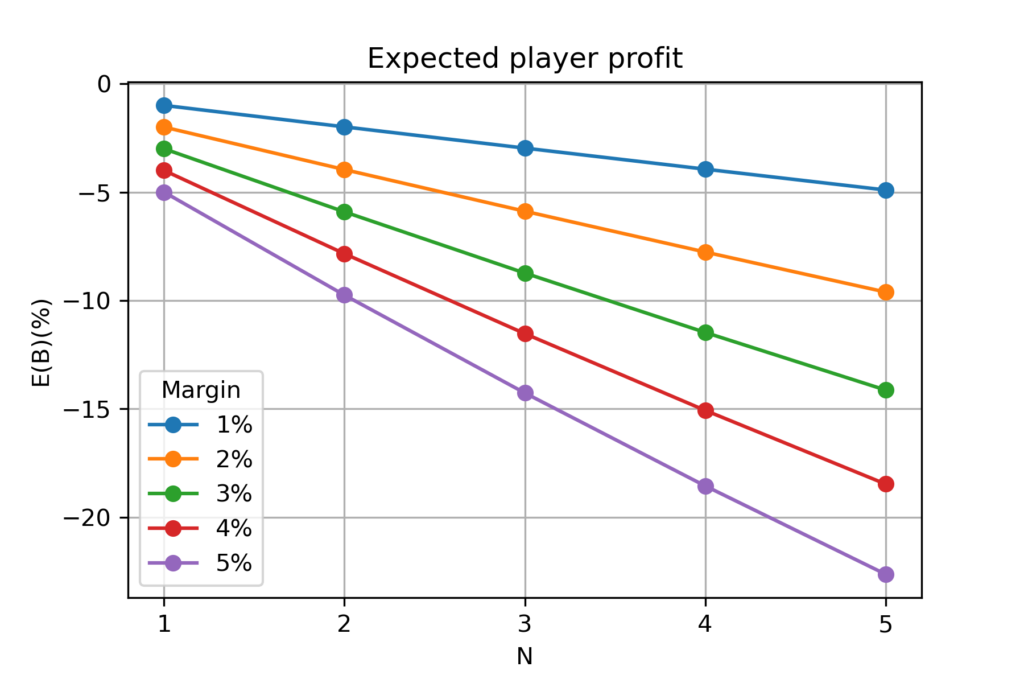# Combined Bets – The Double-Edged Opportunity

### Introduction

After an ultimate math introduction to sport bets: ‘Bookmaking Maths Explained Or In Greed We Trust’, we conclude bookmaking detour with a follow-up dedicated to combined bets.

Combined bets come by many names: accumulator, accumulated bet, parley, AKO, acca, combo, multi bet, etc.

### Definition

In accumulated,  gambler bets on a joint outcome of multiple events rather than a single one. Such combo bet has odds equal to the product of component bets. According to probability laws, the probability of the intersection of independent events is equal to the product of all probabilities.

$P_{12…N} = P_1\times{P_2}\times{…}\times{P_N}=\prod_{i=1}P_i$

, while the odds K offered for such accumulated bet:

$K_{12…N}=\prod_{i=1}\frac{M_i+1}{P_i}$

, where $$M_i$$ is the margin of bet $$i$$ (remember that margins are negative in expressions – player’s perspective, but positive in discussion). We wouldn’t go deeper into variance in margins here, therefore we would assume constant margin M for every bet.

### Expected Profit

Substituting K and P into expected profit formula:

$E(B) = P_{12…N}\times{K_{12…N}} – 1 = (M+1)^N-1$

, assuming that bookmaker predicted real probabilities and did not adjust odds. The margin of accumulated bet propagates with the number of events N. Let’s see how it looks for 1-5% margin and N=1-5.Bookmakers are happy to suffle accumulated bets with N as big as possible. Such combined bets result in much higher interest rate for bookmakers compared with singles. High odds and spectacular winning coupons found on the internet are successful at deceit.

The only reason to play accumulated bets on independent events is having significant advantage over bookmaker in probability assessment and limited amount of money at the same time.

Because of odds adjustment risk (check out the main article), building an accumulated coupon based on ‘sure-bets’ is the worst idea possible, yet very common in practice!

### Hidden Dependencies

Accumulated bets rely on assumption that all components are independent. Therefore bookmakers do not let betting on coupled events; for example Team A wins + Team A scores more than one goal.

Nevertheless, there may be some hidden dependencies between events. Imagine Team A and Team B fighting for the top spot in a football league. Team A is the leader in the table and plays after Team B. Team B is the only contender for championship and needs a win to threat Team A. If Team B loses or draws, then Team A probably would put less effort to the game. For a contrast view, let’s say that:

Team B not wins => Team A loses
Team B wins => Team A wins

Let’s say that Team B has 50% chances to win. Taking two solo bets, one on Team A to win and one on Team B to win:

B not win, A loses => 50%
B wins, A wins => 50%

There is 50% chance for each solo event. Let’s forget margin and taxation right now. Bookmaker offers 2.0 odds for both bets. Net profit from two solo bets is equal to 0.

$E(Solo) = 0.5\times{2}+0.5\times{2}-2 = 0$

$E(Ako) = P_{AB}\times{K_{AB}}-1 = P(B)\times{P(A|B)}\times{K_{AB}}-1$

,where P(A|B) is the conditional probability of A winning if B won. In this example P(A|B)=1, therefore:

$E(Ako) = 0.5\times{2\times{2}}-1 = 1$

Accumulated bet gives 100% profit on average! Strong hidden dependency is a game changer and a field on which unaware bookmaker could get outplayed.

### Final Takeouts

• Margin is the main bet characteristic to deduce from provided odds (bet calculator). Bookmakers who offer low margins should be prefered.
• Casual gambler (not building own statistical system) is supposed to lose money against bookmaker in the long run.
• Taxation factor is often even more impactful than margin.
• Bookmakers could adjust odds to maximize profits. In such case probabilties resolved from odds are false.
• Casual gambler who wants to maximize profit should avoid mainstream bets (i.e. bets on national team) because of risk of playing into adjusted odds.
• There is no ‘safe-bets’; moreover those are susceptible to odds adjustments.
• Playing against odds adjustment is the main win-condition against bookmaker, but assessing how much adjustment is done isn’t easy without statistical system reproducing bookie’s odds.
• If possible, don’t play combo of independent events – the margin propagates with the number of compound bets.
• Finding hidden dependencies gives a chance to achieve profitable accumulated bet.

Thanks for reading! Our short bookmaking tour ends here, hope you learned something useful, maybe more for real life than gambling itself!